Study Materials

# NCERT Solutions for Class 9th Mathematics

Page 3 of 4

## Chapter 4. Linear Equation In Two Variables

### Exercise 4.3

Linear equation in two variable

Excecise : 4.3

1. Draw the graph of each of the following linear equations in two variables:

(i) x + y = 4

(ii) x y = 2

(iii) y = 3x

(iv) 3 = 2x + y

Solution :

(i) x+y = 4   =   y = 4-x(ii) x - y = 2    =  x = 2 + y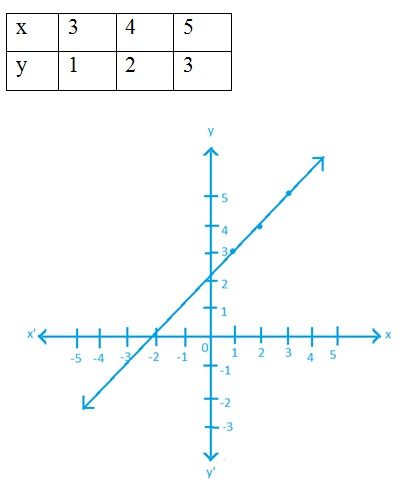(iii) y = 3x  = -3x + y    = y = 3x2. Give the equations of two lines passing through (2, 14). How many more such lines are there, and why?

Solution :   x + y= 16

x - y=  -12

so, infinitely many lines can pass through these lines.

3. If the point (3, 4) lies on the graph of the equation 3y = ax + 7, find the value of a.

Solution :   3y = ax + 7

Putting the value of x and y

3(4) = a(3) +7    =   12 = 3a + 7    =    12 - 7 = 3a4. The taxi fare in a city is as follows: For the first kilo metre, the fare is ` 8 and for the subsequent distance it is ` 5 per km. Taking the distance covered as x km and total fare as Rs y, write a linear equation for this information, and draw its graph.

Solution :  distance covered = x

Total  fare           = y

Then, According To Question

8 + 5(x - 1) = y

= 8 + 5x - 5 = y

= 3 + 5x = y

= 5x -y + 3 = 0

= y = 5x + 3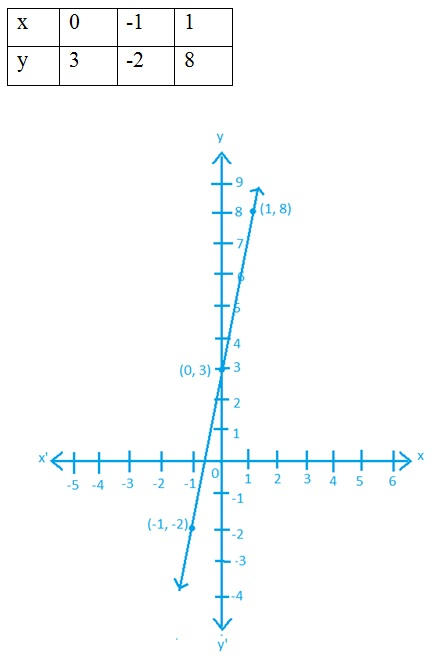5. From the choices given below, choose the equation whose graphs are given in Fig. 4.6 and Fig. 4.7.

For Fig. 4. 6                                 For Fig. 4.7

(i) y = x                                          (i) y = x + 2

(ii) x + y = 0                                  (ii) y = x – 2

(iii) y = 2x                                   (iii) y = –x + 2

(iv) 2 + 3y = 7x                          (iv) x + 2y = 6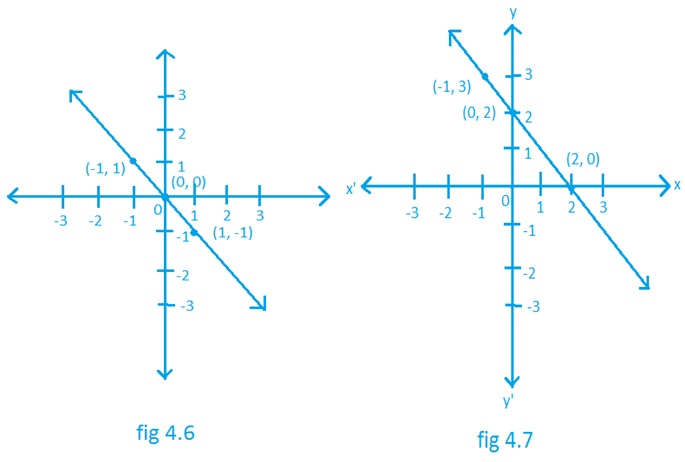Solution :  for fig. 4.6              for fig. 4.7

(ii) x + y = 0              (iii) y = -x + 2

6. If the work done by a body on application of a constant force is directly proportional to the distance travelled by the body, express this in the form of an equation in two variables and draw the graph of the same by taking the constant force as 5 units. Also read from the graph the work done when the distance travelled by the body is :

(i) 2 units                         (ii) 0 unit

Solution :   W= F×S

Constant force= 5 unit

Let the work done be y

Let the distance travelled be x

So,   y = 5x

(i) 2 units

Putting, x = 2

=   y = 5(2)

=   y =  10

(ii) 0 units

Putting, x= 0

=   y = 5(0)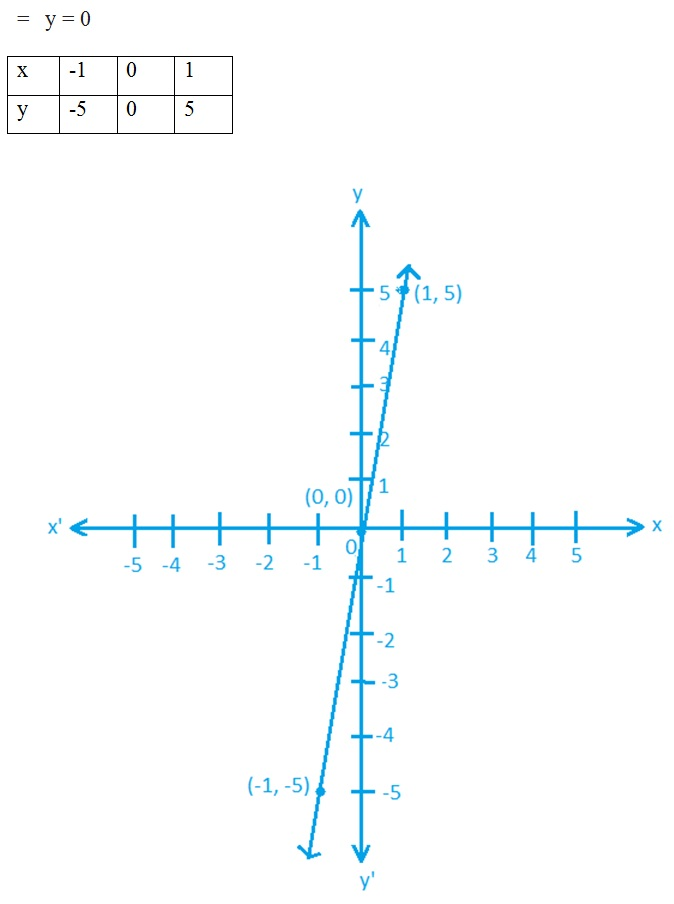7. Yamini and Fatima, two students of Class IX of a school, together contributed 100 towards the Prime Minister’s Relief  Fund to help the earthquake victims. Write a linear equation which satisfies this data. (You may take their contributions as ` x and ` y.) Draw the graph of the same.

Solution :

Together contributed = 100

Contribution by yamini = x

Contribution by fatima= y

According To Question,

x + y= 10

y = 100 - x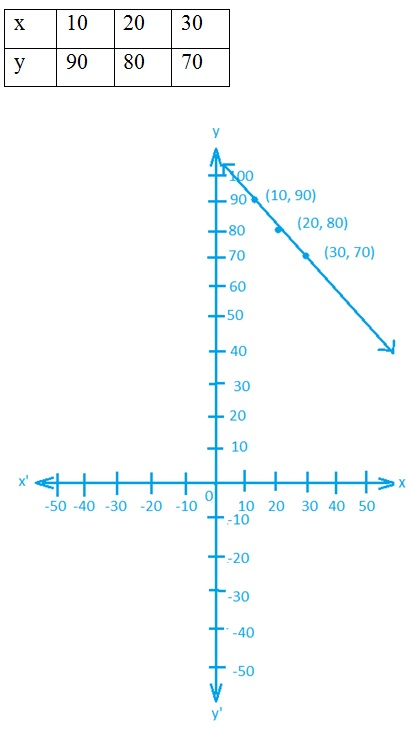8. In countries like USA and Canada, temperature is measured in Fahrenheit, whereas in countries like India, it is measured in Celsius. Here is a linear equation that converts Fahrenheit to Celsius: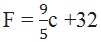(i) Draw the graph of the linear equation above using Celsius for x-axis and Fahrenheit for y-axis.

(ii) If the temperature is 30°C, what is the temperature in Fahrenheit?

(iii) If the temperature is 95°F, what is the temperature in Celsius?

(iv) If the temperature is 0°C, what is the temperature in Fahrenheit and if the temperature is 0°F, what is the temperature in Celsius?

(v) Is there a temperature which is numerically the same in both Fahrenheit and Celsius? If yes, find it.

Solution :Page 3 of 4

Chapter Contents: